CBSE Sample Papers for Class 12 Chemistry Paper 4 are part of CBSE Sample Papers for Class 12 Chemistry Here we have given CBSE Sample Papers for Class 12 Chemistry Paper 4.

## CBSE Sample Papers for Class 12 Chemistry Paper 4

 Board CBSE Class XII Subject Chemistry Sample Paper Set Paper 4 Category CBSE Sample Papers

Students who are going to appear for CBSE Class 12 Examinations are advised to practice the CBSE sample papers given here which is designed as per the latest Syllabus and marking scheme as prescribed by the CBSE is given here. Paper 4 of Solved CBSE Sample Paper for Class 12 Chemistry is given below with free PDF download solutions.

Time Allowed : 3 Hours

Max. Marks : 70

General Instructions

• All questions are compulsory.
• Question number 1 to 5 are very short answer questions and carry 1 mark each.
• Question number 6 to 10 are short answer questions and carry 2 marks each.
• Question number 11 to 22 are also short answer questions and carry 3 marks each.
• Question number 23 is a value based question and carry 4 marks.
• Question number 24 to 26 are long answer questions and carry 5 marks each.
• Use log table, if necessary. Use of calculators is not allowed.

Questions

Question 1.
Name the compound which shows frenkel as well as schottky defect.

Question 2.
Arrange the following according to increasing boiling point: 2-Bromobutane, 2-Bromo-2- methyl propane, 1-Bromopropane and 1-Bromobutane.

Question 3.
A gas is passed through suspension of AgCl in water leads to dissolution of AgCl. Name the gas.

Question 4.
What causes Brownian movement?

Question 5.
Write structure of toluidine.

Question 6.
Give the magnetic behaviour of the following complexes
(a) [CO(NH3)6]3+
(b) [Ni(CN)4]2-

Question 7.
Write the chemical reaction for the following preparations:
(a) Sodium chromate from chromite ore
(b) Potassium permangnate from potassium mangnate
OR
Complete the following chemical equations:

1. MnO4 (aq) + S2O2-3
2. Cr2O2-7 + I $$\underrightarrow { { H }^{ + } }$$

Question 8.
Write the mechanism of the following reaction:
CH2 = CH2 $$\underrightarrow { { dil.H }_{ 2 }{ SO }_{ 4 } }$$ CH3CH2OH

Question 9.
From the given cells:
Ni-Cd cell, Mercury cell, Fuel cell and Dry cell. Answer the following:

1. Which can be used in transistor?
2. Which can be used to produce energy most efficiently?
3. Which cell has longer life?
4. Which cell is used in quartz watches?

Question 10.
Distiniguish between order and molecularity of a chemical reaction.

Question 11.
The rate constant for a first order reaction becomes eight times when the temperature is increased from 360 K to 400 K. Calculate the activation energy for the reaction.

Question 12.
Complete the following reactions:Question 13.
An element crystallises in f.c.c. lattice with cell edge of 250 pm. Calculate the density of 300 g of this element that contains 2 x 1024 atoms.

Question 14.
Explain the following terms:

1. Electrophoresis
2. Dialysis
3. Tyndall effect

Question 15.
Give reasons for:

1. Cd2+ salts are white in colour.
2. Out of Mn3+ and Cr3+, Mn3+ is a stronger oxidising agent.
3. Actinoids are known as rare earth elements.

Question 16.
Carry out the following conversions:
(a) Benzene to m-nitro acetophenone
(b) Benzaldehyde to benzophenone
(c) Propanone to propene

Question 17.

1. Why is froth floatation process selected for the concentration of sulphide ores?
2. What is the role of NaCN in froth floatation process?
3. Discuss the role of SiO2 in extraction of Cu from copper matte?

Question 18.
Write the Nemst equation and calculate the emf of the following cell at 298 K.
Sn/Sn2+ (0.050 M) || H+(0.020 M) | H2(s) (1 bar) | Pt(s)Question 19.

1. Name the water soluble component of starch.
3. Name an amine hormone

Question 20.

1. How is double salt different from co-ordination compounds?
2. What are primary and secondary valencies according to Werner’s theory?
3. Write the IUPAC name for ionisation isomer of [Co(CH3NH2)2(NH3)3Br] SO4.

Question 21.
Give the structure of A, B and C in the following reactions: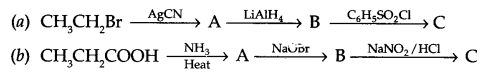Question 22.
(a) Write the monomer of
(i) Glyptal
(ii) Neoprene
(b) Arrange the following according to increasing intermolecular forces: Elastomers, fibres, thermoplastics.

Question 23.
Shilpa, a domestic helper of Mrs. Reeta, had a wound on her leg but she was negligent for the same. Reeta applied dettol on her wound and asked her to go to the doctor if it does not get cured. Answer the following questions:

1. What type of substance can be used for external injuries (like cuts, currents etc.)?
2. Name the components of dettol.
3. Name two more substance used for the same purpose.
4. What are values displayed by Mrs. Reeta?

Question 24.
(a) Why is there a larger difference in the boiling points of butanal and butan-l-ol?
(b) Distinguish between
(i) Ethanal and propanal
(ii) methanamine and N-methylmethanamine
(c) Arrange according to the increasing order of their acidic strength:
C6H5COOH, FCH2COOH, NO2CH2COOH
(d) Carboxylic acid does not give nucleophilic addition reaction like carbonyl compounds. Explain.

OR
An organic compound having molecular formula (C9H10O) forms 2, 4-DNP derivative, reduces tollen’s reagent and undergoes cannizaro reaction. On vigrous oxidation it gives 1, 2, 4 benzene tricarboxylic acid. Identify the compound and write all reactions involved in this process.

Question 25.
(a) When 1.92 g of sulphur was dissolved in 100 grams of CS2, the freezing point will be lowered by 0.383 K. Calculate the formula of Sx associated in CS2.
(Kf for CS2 = 3.83 K kg mol-1, Atomic mass of S = 32 g mol-1)
(b) Define Van’t Hoff factor. What is its value for dimerisation of ethanoic acid in benzene?

OR
The vapour pressures of pure liquids ‘A’ and ‘B’ are 450 and 700 mm. Hg at 350 K respectively. Find out the composition of the liquid mixture if total vapour pressure is 600 mm Hg. Also find the composition of the vapours of these liquids in vapour phase.

Question 26.
(a) Account for the following:
(i) H3PO3 is a diprotic acid
(ii) Decomposition of O3 molecule is a spontaneous process
(iii) Noble gases have low boiling point.
(b) Draw the structure of
(a) XeF4
(b) PCl4
OR

1. Why are halogens coloured?
2. What inspired M. Bartlett for carrying out reactions with xenon?
3. Arrange the following in the order of property indicated against each set:
1. HF, HCl, HBr, HI (Increasing B. Pt)
2. H3PO2, H3PO4, H3PO3 (Increasing acidic nature)
3. NH3, PH3, AsH3 (Increasing Bond angle)

AgBr.

1-Bromopropane < 2-Bromo-2-methyl propane < 2-Bromopropane < 1-Bromobutane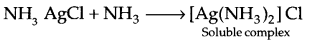Unbalanced bombardment of particles of dispersion medium with particles of dispersed phase causes Brownian movement.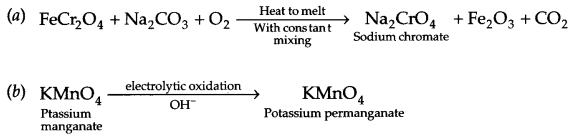OR

1. MnO4 + S2O2-3 → MnO2 + SO2-4 + 2OH
2. Cr2O2-7 + I $$\underrightarrow { { H }^{ + } }$$ Cr3+ + I2 + H2O

H2SO4 + H2O → H3O+ + HSO4
Protonation of ethene1. Dry cell
2. Fuel cell
3. Ni-Cd cell
4. Mercury cell

 Order Molecularity (i) It is the sum of powers of all the reactants present in the rate law equation. (i) It is the sum of powers of all the reactants present in the law of mass action equation. (ii) It is based on experiment. (ii) It is based on balanced chemical reaction. (iii) It can be zero, fraction, positive and a negative integer (iii) It cannot be zero or fraction, only positive integral value is possible. (iv) Applicable for all types of reactions. (iv) Applicable only for elementary reactions.

If rate constant at 360 K is K, then at 400 K it will be 8K.
From Arrhenius equation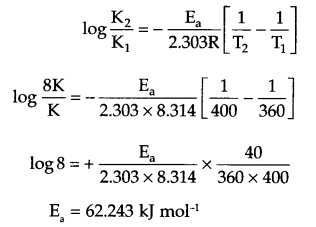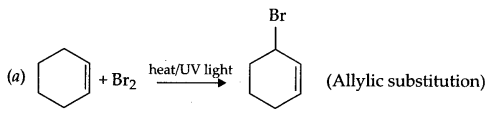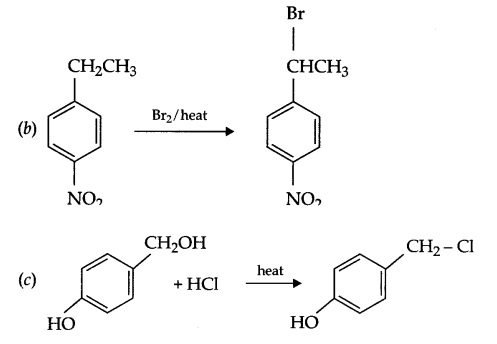From question:
Z = 4
a = 250 pm = 250 × 10-10 cm
mass of sample = 300 g
Number of particles in sample = 2 × 1024 atoms
Since d = $$\frac { Zm }{ { a }^{ 3 }n }$$
where m = mass of sample, n = no. of particles in the sampled = 38.4 g/cm3

1. Electrophoresis : The movement of colloidal particles towards oppositely charged electrode in an electric field is called electrophoresis.
2. Dialysis : It is the process of eliminating dissolved substances from a colloidal solution by means of diffusion through a suitable membrane.
3. Tyndall effect : When a beam of light is passed through a colloidal solution placed in dark, the path of light becomes visible on viewing from right angle to the direction of passage of light. It is due to scattering of light by particles of dispersion phase and this effect is known as Tyndall effect.

1. Cd2+ ion posses 4d10 electronic configuration it means there is no unpaired electron and due to this Cd2+ salts are white in colour.
2. Mn2+ has 3d5, half filled stable electronic configuration. As a result Mn3+ acts as a stronger oxidising agent than Cr3+ which has half filled f configuration which makes Cr3+ more stable and it does not act as an oxidising agent.
3. Other than first five elements belonging to actinoide series all are prepared in laboratory by nuclear transmutation, so they are known as rare earth elements.1. Sulphide ores are preferentially wetted by oil and easily come out as froth, so froth floatation process is used for such ores.
2. NaCN prevents the formation of undesirable froth, hence it acts as a depressent during froth floatation process.
For example, during the concentration of PbS, ZnS is prevented to come as the froth by NaCN by making a complex.
NaCN + ZnS → Na2[ZnCN)4] + Na2S
3. SiO2 combines with FeO present in copper matte to form the slag.
FeO + SiO2 → FeSiO3

For the given cell
Reaction at anode: Sn → Sn2+ + 2e
Reaction at cathode: 2H+ + 2e → H2(g)
Net reaction: Sn(s) + 2H+(aq) → Sn2+(aq) + H2(g)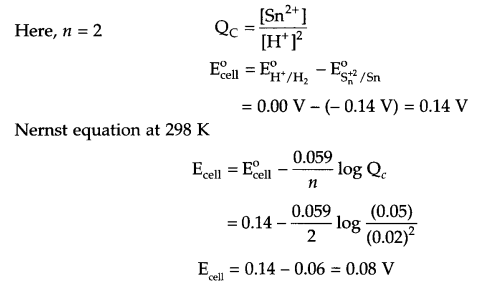1. Amylase is the water soluble part of starch. (It is a straight chain polymer of a-glucose attached with a-glycosidic linkage)
2. The amino acids are linked with one another through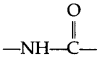3. Thyroxine

1. Double salts as well as co-ordination compounds are formed by the combination of two or more stable compounds in stoichiometric ratio. But double salt get dissociated into constituent simple ions completely when dissolved in water. On the other hand complex ions present in co-ordination compounds do not get dissociated into constituent ions. Examples- double salt- Mohr salt, Potash atom
Complexes – [Fe(CN)6]4 and K2[PtCl6]
2. Ionisable valencies are normally known as primary valency. On the other hand non ionisable valencies are known as secondary valencies.
3. Ionisation isomer of given complex is [Co(CH3NH2) (NH3)3Br] SO4
IUPAC Name : Triammine dimethanminebromocobalt (III) sulphate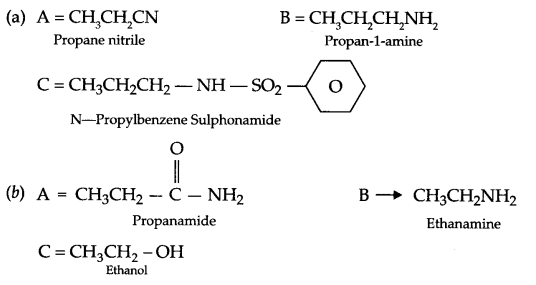(a) (i) Glyptal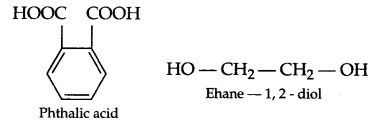(ii) Neoprene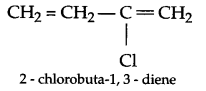(b) Elastomers < Thermoplastics < fibres

1. Antiseptics
2. Chloroxylenol, pine oil, isopropanol, castor oil, soap and water.
3. Iodoform, Tincture of iodine (2-3% I2 solution in ethanol-water mixture), soframycine, furacine etc. (any two)
4. Concern for others, knowledge of chemistry, helping and advising nature, equality for all etc.

(a) There is intermolecular hydrogen bonding in butan-1-ol which is a stronger attracting force than simple dipole-dipole interatomic interactions of butanal. Hence boiling point of butanol is much higher.
(b) (i)

 Reagent Ethanal Propanal NaOH + I2 heat Yellow ppt. of CHI3 will form No such precipitation

(ii)

 Reagent Methanamine N-methylmethanamine CHCl3 + KOH Heat Foul smelling substance will form No such smell

(c) C6H5COOH < NO2CH2COOH < FCH2COOH (acidic strength)
(d) In carboxylic acids there is no carbonyl group like aldehydes and ketones, carboxylic group is altogether a different group due to the following resonance structures.OR
Since compound (C4H10O)gives 2, 4-DNP derivative and reduces tollen’s reagent, it means it is an aldehyde. On vigrous oxidation it gives 1, 2, 4 benzene tricarboxylic acid, it means other than CHO group there will be two alkyl (methyl) groups on 2 and 4 position w.r.t. CHO on benzene ring. So, the given compound is: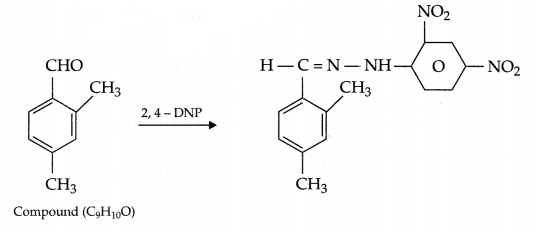(a) mass of solute (wB) = 1.92 g, mass of solvent (wA) = 100 g
∆Tf = 0.383 K
Kf = 3.83 K Kg mol-1 for CS2
To find the formula of S
So the formula of sulphur will be Sx.(b) Van’t Hoff factor: It is represented by i and it is to account for the extent of dissociation or association of a solute in a solution. This factor i is defined as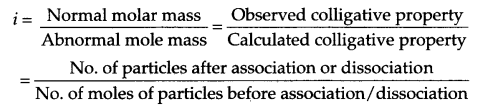For ethanoic acid in benzene:
i = $$\frac { 1 }{ 2 }$$

OR
From question
PA° = 450 mm Hg,
PB° = 700 mm Hg
PTotal = 600 mm Hg
It is to be found that xA = ?, xB = ?
If mol fraction in vapour phase is yA and yB respectively, they also need to be found,
From Raoult’s law(a) (i) H3PO3 posses 2 OH groups, so it is diprotic(iii) Among noble gas molecules, there is weak intermolecular van der waals forces only.OR

1. Halogens absorb light in visible region, hence they are coloured.
2. On the basis of his experiment of O2 and PtF6 in which O2 form 02+ ions in which ionisation energy of O2 equal to 1175 kJ/mol, hence same reaction may be possible with xenon having ionisation energy 1170 kJ. This thought inspired N. Bartlett to carry out experiments between Xe and PtF6 to form Xe[PtF6].
Xe + PtF6 → Xe [PtF6]
1. HCl < HBr < HI < HF
2. H3PO4 < H3PO3 < H3PO2
3. ASH3 < PH3 < NH3

We hope the CBSE Sample Papers for Class 12 Chemistry Paper 4 help you. If you have any query regarding CBSE Sample Papers for Class 12 Chemistry Paper 4, drop a comment below and we will get back to you at the earliest.